# How To Compare Two Dataframes with Pandas compare?

• Last Updated : 12 Nov, 2020

A DataFrame is a 2D structure composed of rows and columns, and where data is stored into a tubular form. It is mutable in terms of size, and heterogeneous tabular data. Arithmetic operations can also be performed on both row and column labels.

To know more about the creation of Pandas DataFrame.

Here, we will see how to compare two DataFrames with pandas.DataFrame.compare.

Syntax:

DataFrame.compare(other, align_axis=1, keep_shape=False, keep_equal=False)

So, let’s understand each of its parameters –

1. other : This is the first parameter which actually takes the DataFrame object to be compared with the present DataFrame.
2. align_axis : It deals with the axis(vertical / horizontal) where the comparison is to be made(by default False).0 or index : Here the output of the differences are presented vertically, 1 or columns : The output of the differences are displayed horizontally.
3. keep_shape : It means that whether we want all the data values to be displayed in the output or only the ones with distinct value. It is of bool type and the default value for it is “false”, i.e. it displays all the values in the table by default.
4. keep_equal : This is mainly for displaying same or equal values in the output when set to True. If it is made false then it will display the equal values as NANs.

Returns another DataFrame with the differences between the two dataFrames.

Before Starting, an important note is the pandas version must be at least 1.1.0.

To check that, run this on your cmd or Anaconda navigator cmd.

```import pandas as pd
print(pd.__version__)

```

If it is 1.1.0 or greater than that, you are good to go! Otherwise, you can install pandas compatible version by the command into your window cmd by running as an administrator, or otherwise into your Anaconda navigator if it is added to the path.

```# if you want the latest version available

# or if you want to specify the version
pip install pandas==1.1.0
```

Implementation:

Step 1: we will create our first dataframe.

Approach:

1. Import pandas for DataFrame
2. Import NumPy for any NAN values which can come up through operations or insert
3. Create the DataFrames using pandas.DataFrame and passing the value for your rows, columns
4. Specify the column headings(from the value you have passed in the dictionary)

## Python3

 `# pandas version == 1.1.0 (min)``import` `pandas as pd``import` `numpy as np`` ` `# create your first DataFrame``# using pd.DataFrame``first_df ``=` `pd.DataFrame(``    ``{``        ``"Stationary"``: [``"Pens"``, ``"Scales"``,``                       ``"Pencils"``, ``"Geometry Box"``,``                       ``"Crayon Set"``],``        ``"Price"``: [``100``, ``50``, ``25``, ``100``, ``65``],``        ``"Quantity"``: [``10``, ``5``, ``5``, ``2``, ``1``]``    ``},``    ``columns``=``[``"Stationary"``, ``"Price"``, ``"Quantity"``],``)``# Display the df``first_df`

Output: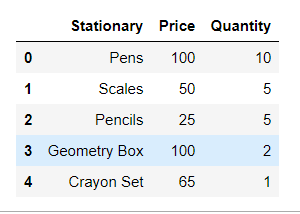Step 2:Now, let’s make out the next DataFrame for comparing their values:

## Python3

 `# creating the second dataFrame by ``# copying and modifying the first DataFrame``second_df ``=` `first_df.copy()`` ` `# loc specifies the location,``# here 0th index of Price Column``second_df.loc[``0``, ``'Price'``] ``=` `150` `second_df.loc[``1``, ``'Price'``] ``=` `70``second_df.loc[``2``, ``'Price'``] ``=` `30``second_df.loc[``0``, ``'Quantity'``] ``=` `15``second_df.loc[``1``, ``'Quantity'``] ``=` `7``second_df.loc[``2``, ``'Quantity'``] ``=` `6`` ` `# display the df``second_df`

Output:We are creating another DataFrame by copying the table structure of the first_DataFrame with certain modifications. Now, let’s see the content of the second_DataFrame

Step 3: let us do our main operation – compare.

Here we have performed two operations, firstly to align the differences of the changes in the columns, for which the align_axis by default is set to 1 and table will be with columns drawn alternately from self and other.

## Python3

 `#Align the differences on the columns``first_df.compare(second_df)`

Output: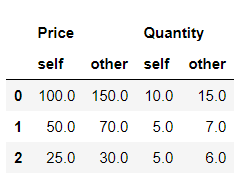And secondly, we have set the align_axis = 0 which makes the table rows drawn alternately from self and others.

## Python3

 `# align the differences on rows``first_df.compare(second_df,align_axis``=``0``)`

Output: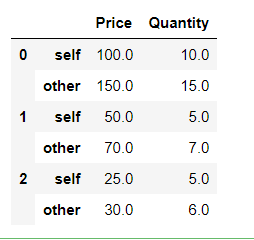Step 4: let’s try to set equal values as true and false.

If keep_equal is true, the result also keeps values that are equal. Otherwise, equal values are shown as NaNs. By default it is set to False.

## Python3

 `# Keep the equal values``first_df.compare(second_df, keep_equal``=``True``)`

Output: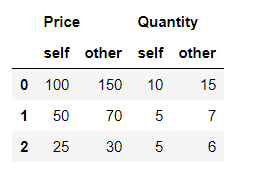## Python3

 `# Keep the equal values False``first_df.compare(second_df, keep_equal``=``False``)`

Output: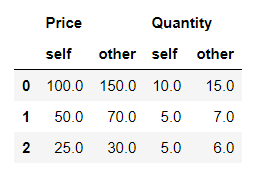Step 5: Let’s now check the keep_shape which is by default false. If it is set to true then, all the rows and columns are present in the table, or else only the ones with distinct values are kept.

## Python3

 `#Keep all original rows and columns``first_df.compare(second_df,keep_shape``=``True``)`

Output: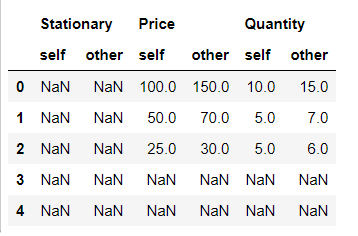## Python3

 `#Keep all original rows and columns and`` ``#also all original values``first_df.compare(second_df,keep_shape``=``True``, keep_equal``=``True``) `

Output: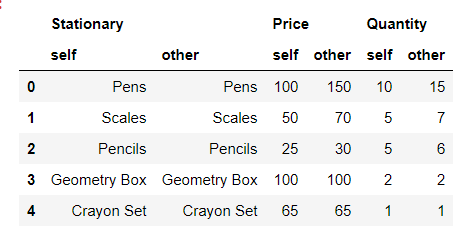Here the keep_shape true will keep the structure and will set all the unchanged values to nan. Whereas, the keep_shape and keep_equal true will keep the whole structure of the table as well as also the values which are not changed

Note: Before comparing two DataFrames make sure that the number of records in the first DataFrame matches the number of records in the second DataFrame.  If not so, you will be getting a value error which is :

ValueError: Can only compare identically-labeled Series objects

My Personal Notes arrow_drop_up